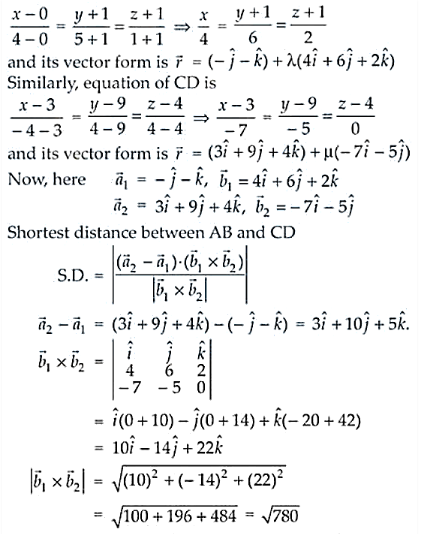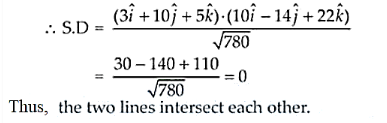# Prove that the line through A (0, –1, –1) and B (4, 5, 1)`
Question:

Prove that the line through A (0, –1, –1) and B (4, 5, 1) intersects the line through C (3, 9, 4) and D (– 4, 4, 4).

Solution:

Given points, A (0, –1, –1) and B (4, 5, 1)

C (3, 9, 4) and D (– 4, 4, 4).

Cartesian form of equation AB is